177
Created byMaster Student

Chemistry Practice Test #4

1 / 30

A 2.35-gram sample was dissolved in water, and the chloride ions were precipitated by adding silver nitrate (Ag+ + Cl- → AgCl). If 0.435 g of precipitate was obtained, what is the percentage of chlorine in the sample?

2 / 30

Which of the following is expected to have the largest bond polarity?

3 / 30

The equilibrium constant of a certain reaction is 2.6 × 108 at 25 °C. What is the value of ΔG°?

4 / 30

Under which conditions will a real gas most closely behave as an ideal gas?

5 / 30

Approximately how many half-lives will it take to reduce 10.0 kg of a radioactive substance to 1.0 microgram of that substance?

6 / 30

Which of the following has an octet of electrons around the central atom?

7 / 30

What is the conjugate acid of the H2PO4- ion?

8 / 30

Balance the following skeleton reaction in acid solution using the ion-electron method to obtain a reaction with the smallest possible whole-number coefficients. The sum of all the coefficients in the balanced equation is

IO3- + Cl- → ClO- + I2

9 / 30

Hund's rule requires that

10 / 30

The rate law for the reaction of 2 A + B → 2 P is

11 / 30

In acid solution the bromate ion, BrO3- can react with other substances, resulting in Br2. Balance the half-reaction for bromate ions forming bromine. The balanced half-reaction has

12 / 30

Given the two standard reduction equations and their potentials below, write the thermodynamically favoured chemical reaction and its standard cell potential.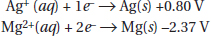A.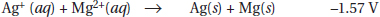B.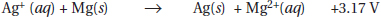C.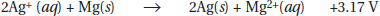D.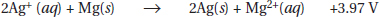13 / 30

Which of the following is a balanced chemical reaction?

14 / 30

Which of the following geometries corresponds to a substance that has five sigma bonds and one nonbonding pair of electrons?

15 / 30

An ideal solution is a

16 / 30

How many millilitres of 0.250 M KOH does it take to neutralize completely 50.0 mL of 0.150 M H3PO4?

17 / 30

The collision theory of reaction rates does not include

18 / 30

The symbol for antimony is

19 / 30

What is the pH of a solution made by dissolving 0.0300 mol of sodium ethanoate in enough water to make 50 mL of solution (Ka for ethanoic acid is 1.8 × 10-5)?

20 / 30

A 50.0 mL sample of 0.0025 M HBr is mixed with 50.0 mL of 0.0023 M KOH. What is the pH of the resulting mixture?

21 / 30

When an ideal gas is allowed to expand isothermally, which one of the following is true?

22 / 30

The quantum number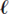signifies the

23 / 30

The weight percent of sodium hydroxide dissolved in water is 50%. What is the mole fraction of sodium hydroxide?

24 / 30

What is the molecular mass of a gas that has a density of 2.05 g/L at 26.0 °C and 722 torr?

• A.
• B.
• C.
• D.

25 / 30

Which of the following is a reduction half-reaction?

1. Cu2+ + e- → Cu+

2. Cu+ + e- → Cu0

3. Fe2+ → Fe3+ + e-

26 / 30

The reaction of Br2(g) with Cl2(g) to form BrCl(g) has an equilibrium constant of 15.0 at a certain temperature. If 10.0 grams of BrCl is initially present in a 15.0-litre reaction vessel, what will the concentration of BrCl be at equilibrium?

27 / 30

Each resonance form of the nitrate ion, NO3-, has how many sigma and how many pi bonds?

28 / 30

Twenty-five milligrams of sucrose (C12H22O11) is dissolved in enough water to make 1.00 litre of solution. What is the molality of the solution?

29 / 30

Which of the following is considered a metalloid?

30 / 30

Potassium-40 is a minor isotope found in naturally occurring potassium. It is radioactive and can be detected on simple radiation counters. How many protons, neutrons, and electrons does potassium-40 have when it is part of K2SO4?